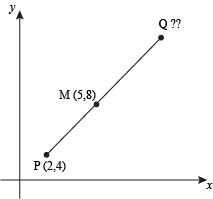# Find the other end point of a line with given one end and mid

Given a midpoint of line(m1, m2) and one coordinate of a line (x1, y1), find the other end point(x2, y2) of a line

Examples:

```Input  : x1 = –1, y1 = 2, and
m1 = 3, m2 = –6
Output : x2 = 7, y2 = 10

Input  : x1 = 6.4, y1 = 3 and
m1 = –10.7, m2 = 4
Output : x2 = 3, y2 = 4
```

## Recommended: Please try your approach on {IDE} first, before moving on to the solution.The Midpoint Formula: The midpoint of two points, (x1, y2) and (x2, y2) is the point M found by using:
M=((x1+x2)/2, (y1+y2)/2),
We have need of a (x2, y2) so we modifies the formula

```          m1 = ((x1+x2)/2),  m2 = ((y1+y2)/2)
2*m1 = (x1+x2),  2*m2 = (y1+y2)
x2 = (2*m1 - x1),  y2 = (2*m2 - y1)
```

## C++

 `// CPP program to find the end point of a line ` `#include ` `using` `namespace` `std; ` ` `  `// CPP function to find the end point of a line ` `void` `otherEndPoint(``int` `x1, ``int` `y1, ``int` `m1, ``int` `m2) ` `{ ` `    ``// find end point for x cordinates ` `    ``float` `x2 = (``float``)(2 * m1 - x1); ` ` `  `    ``// find end point for y cordinates ` `    ``float` `y2 = (``float``)(2 * m2 - y1); ` ` `  `    ``cout << ``"x2 = "` `<< x2 << ``", "` `         ``<< ``"y2 = "` `<< y2; ` `} ` ` `  `// Driven Program ` `int` `main() ` `{ ` `    ``int` `x1 = -4, y1 = -1, m1 = 3, m2 = 5; ` `    ``otherEndPoint(x1, y1, m1, m2); ` `    ``return` `0; ` `} `

## Java

 `// Java program to find the end point of a line ` `class` `GFG { ` `     `  `    ``// CPP function to find the end point ` `    ``// of a line ` `    ``static` `void` `otherEndPoint(``int` `x1, ``int` `y1,  ` `                               ``int` `m1, ``int` `m2) ` `    ``{ ` `        ``// find end point for x cordinates ` `        ``float` `x2 = (``float``)(``2` `* m1 - x1); ` `     `  `        ``// find end point for y cordinates ` `        ``float` `y2 = (``float``)(``2` `* m2 - y1); ` `     `  `        ``System.out.println(``"x2 = "` `+ x2 + ``", "` `                               ``+ ``"y2 = "` `+ y2); ` `    ``} ` `     `  `    ``// Driven Program ` `    ``public` `static` `void` `main(String args[]) ` `    ``{ ` `        ``int` `x1 = -``4``, y1 = -``1``, m1 = ``3``, m2 = ``5``; ` `        ``otherEndPoint(x1, y1, m1, m2); ` `    ``} ` `} ` ` `  `// This code is contributed by JaideepPyne. `

## Python3

 `# Python3 program to find the end ` `# point of a line ` ` `  `# function to find the end point ` `# of a line ` `def` `otherEndPoint(x1, y1, m1, m2): ` `     `  `    ``# find end point for x cordinates ` `    ``x2 ``=` `(``2` `*` `m1 ``-` `x1) ` ` `  `    ``# find end point for y cordinates ` `    ``y2 ``=` `(``2` `*` `m2 ``-` `y1) ` ` `  `    ``print` `(``"x2 = {}, y2 = {}"`  `               ``. ``format``(x2, y2)) ` ` `  `# Driven Program ` `x1 ``=` `-``4` `y1 ``=` `-``1` `m1 ``=` `3` `m2 ``=` `5` `otherEndPoint(x1, y1, m1, m2) ` ` `  `# This code is contributed by  ` `# Manish Shaw (manishshaw1) `

## C#

 `// C# program to find the ` `// end point of a line ` `using` `System; ` ` `  `class` `GFG { ` `     `  `    ``// function to find the  ` `    ``// end pointof a line ` `    ``static` `void` `otherEndPoint(``int` `x1, ``int` `y1,  ` `                              ``int` `m1, ``int` `m2) ` `    ``{ ` `         `  `        ``// find end point for x cordinates ` `        ``float` `x2 = (``float``)(2 * m1 - x1); ` `     `  `        ``// find end point for y cordinates ` `        ``float` `y2 = (``float``)(2 * m2 - y1); ` `     `  `        ``Console.WriteLine(``"x2 = "` `+ x2 + ``", "` `                          ``+ ``"y2 = "` `+ y2); ` `    ``} ` `     `  `    ``// Driver Program ` `    ``public` `static` `void` `Main(String []args) ` `    ``{ ` `        ``int` `x1 = -4, y1 = -1, m1 = 3, m2 = 5; ` `        ``otherEndPoint(x1, y1, m1, m2); ` `    ``} ` `} ` ` `  `// This code is contributed by nitin mittal. `

## PHP

 ` `

Output:

```x2 = 10, y2 = 11
```

GeeksforGeeks has prepared a complete interview preparation course with premium videos, theory, practice problems, TA support and many more features. Please refer Placement 100 for details

My Personal Notes arrow_drop_upCheck out this Author's contributed articles.

If you like GeeksforGeeks and would like to contribute, you can also write an article using contribute.geeksforgeeks.org or mail your article to contribute@geeksforgeeks.org. See your article appearing on the GeeksforGeeks main page and help other Geeks.

Please Improve this article if you find anything incorrect by clicking on the "Improve Article" button below.

Article Tags :
Practice Tags :

Be the First to upvote.

Please write to us at contribute@geeksforgeeks.org to report any issue with the above content.